# Plasma Equilibrium

The unperturbed (by the tearing mode) plasma equilibrium is such that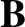(5.18)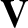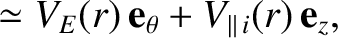(5.19)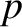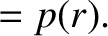(5.20)

(See Sections 2.11, 2.24, and 3.2.) Here,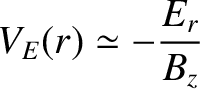(5.21)

is the (dominant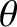-component of the) E-cross-B velocity [see Equation (2.138)], and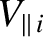the ion parallel fluid velocity.

Now, the resonant layer is assumed to have a radial thickness that is much smaller than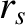. (See Section 5.5.) Hence, we only need to evaluate plasma equilibrium quantities in the immediate vicinity of the rational surface. Equations (4.1), (4.23), (4.24), and (5.1)–(5.4) imply that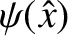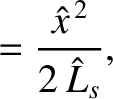(5.22)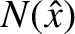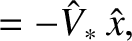(5.23)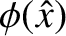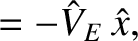(5.24)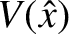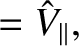(5.25)

where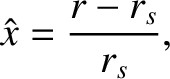(5.26)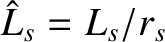,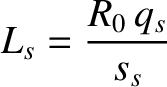(5.27)

is the magnetic shear length,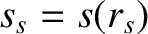,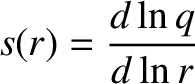(5.28)

the magnetic shear,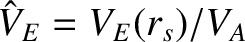,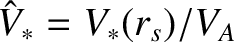,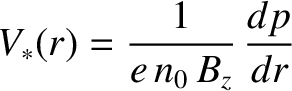(5.29)

the (dominant-component of the) diamagnetic velocity [see Equation (4.11)], and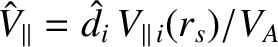. We also have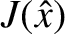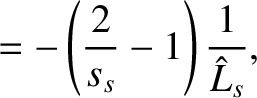(5.30)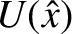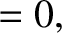(5.31)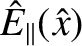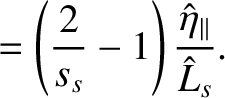(5.32)

Note that we are neglecting any radial gradients in the equilibrium MHD fluid velocity because, in conventional tokamak plasmas, such gradients do not significantly affect the linear response of a resonant layer, due to the fact that the gradients are comparatively weak (i.e.,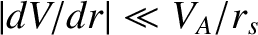) combined with the fact that a linear resonant layer is very narrow . (See Section 5.5.)